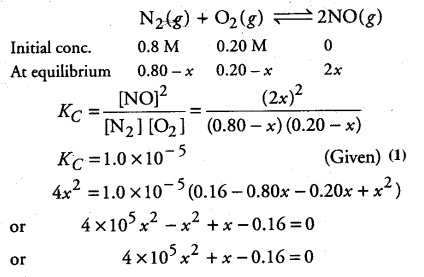# Calculate the equilibrium concentration of the reactants

The reaction, \${ N }_{ 2 }\$(g) + 02(g)----------> 2NO(g) contributes to air pollution wherever a fuel is burnt in air at a high temperature. At 1500 K, equilibrium constant K for it, is 1.0 x 10-5. Suppose in a case [N2]= 0.80 mol L-1 and= 0.20 mol L-1 before any reaction occurs. Calculate the equilibrium concentration of the reactants and the product after the mixture has been heated to 1500 K.